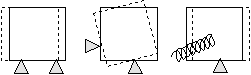﻿ 20-sim webhelp > Library > Iconic Diagrams > Mechanical > Translation > 2DSmallRotation > TwoDPoint Models

# TwoDPoint Models

Navigation:  Library > Iconic Diagrams > Mechanical > Translation > 2DSmallRotation >

# TwoDPoint ModelsTwoDPoint models are used to connect 1D models to TwoDBodies. They indicate a 1D force or torque that is applied in a certain direction, onto a body.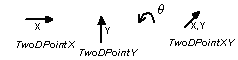A point model forms the connection between the single degree of freedom part of a system and the center of mass of a body. Consider the example below, where force F in x-direction (single degree of freedom) is pushing upon a body (three degrees of freedom) at an offset YP from the body center of mass.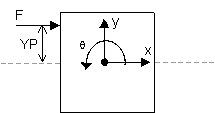To create such a construct, the TwoDPoint-X model is used: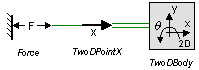The TwoDPoint-X model describes the translation of a 1D force (x-direction) to a 2D-force (x-, y- and q-direction) and vice versa the translation of a 2D-velocity (x-, y- and q-direction) to a 1D velocity (x-direction).

There are four point models. Three point models transform from x-direction, y-direction and q-direction to 2D. One point models transforms from direction with arbitrary orientation to 2D.

## Fixation of bodies

If a TwoDBody model is used in combination with TwoDPoint models, at least three non-coinciding, unequal (i.e. not having the same offsets) TwoDPoints must be connected to prevent the body from free motion. This is obvious when we interpret the point models physically. Point models can be interpreted as constraints on motion in one direction. The motion in the other two directions is free.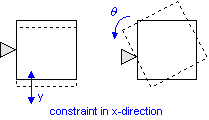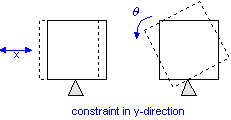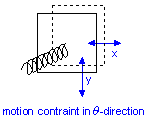To show that two points are not sufficient to constrain all motions look at the figure below.